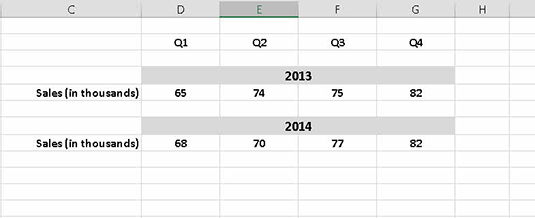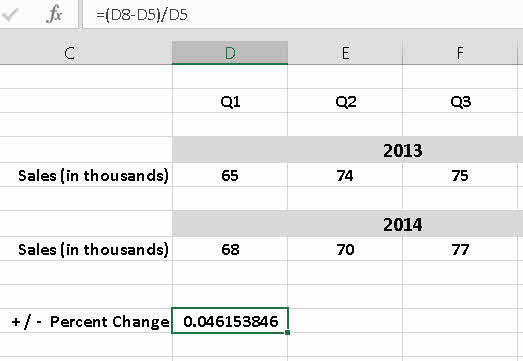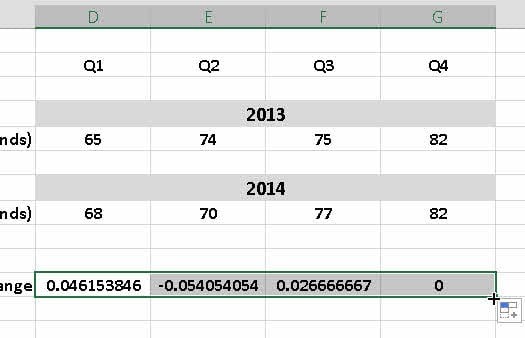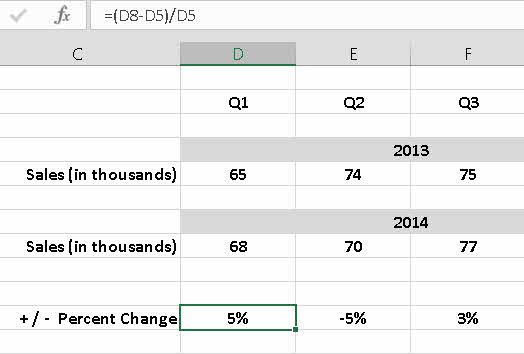##### Statistical Analysis with Excel For DummiesA common need in business and when working with Excel is calculating the percentage a value changes from one period to another. For example, showing how revenue changed from one quarter of the current year to the same quarter of the previous year is a standard metric reported in business. Excel is the perfect platform for working with this standard business formula.

The formula for calculating change is (new value – old value) / old value. That is, first calculate the difference between the values by subtracting the old value from the new value. Then divide that result by the old value. Fire up Excel, and give it a try!

1. Enter some numbers in a worksheet.

This example uses sales figures covering two years, broken out by quarters. One year follows the other in a vertical perspective.2. Enter a summary row.

In this example, the row is below the data. A formula is entered in the column for the first quarter. The formula in cell D11 is

=(D8-D5)/D5Make sure to put parentheses around the subtraction operation; otherwise, the result will be incorrect. Also, make sure that the cell is formatted to General and the Show Formulas button in the Formulas tab is not clicked. If it is, Excel shows you the formula and not the results of the calculation. Frustrating. But it is what it is.

3. Using the drag handle (lower-right corner of the cell), drag across the row to enter the formula in the three columns for the second, third, and fourth quarter. After copying the formula to the adjacent cells, click each cell and then click the fx button in the formula bar and then click the green check mark to commit the change. If you do not do this, Excel will copy the value from the first cell to the last and not recalculate.The calculated value can be positive, negative, or zero. A positive number shows a gain, or increase. A negative number shows a loss, or decrease. A result of zero indicates no change.

A value returned from this formula is a percentage and often appears as a fraction. If the percent change is greater than 100, the value will be greater than 1 (or greater than –1 for a loss of more than 100 percent).

4. Change the formatting to a percentage.

Excel’s formatting options includes a handy button that multiplies by 100 and puts the percentage sign (%) in the result. With the cells containing the formulas selected, click the disclosure button on the Number Format box on the Home tab of the Ribbon; then, choose Percentage from the drop-down list.The change in a value over time is best presented as a percentage. Just showing the difference as a number does not give it a conclusive meaning. Saying that sales have increased by 100 units does not make it clear whether this news is good or bad. Saying that sales increased by 10 percent is clearly good news.

5. Review your worksheet to ensure the calculated results appear as percentages.

For all the great number-crunching you do, when you hand the worksheet up to management, it should be dressed to the nines. On the worksheet it is now uber-easy to see the percentage change in the cells along Percent Change (+/–) row.